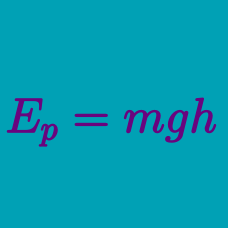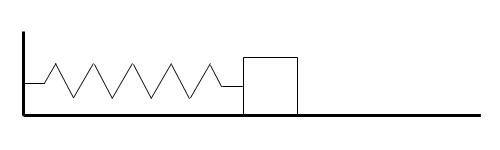Classical Mechanics

# Conservative vs. non-conservative forcesAs shown in the figure above, a spring is attached horizontally to a vertical wall, and an object lies on a frictionless horizontal floor, attached to the other end of the spring. A man pulls the object so that the spring doubles in length, and then let the object go. Which of the following statements is/are true concerning this situation?

I. The force exerted by the spring on the object is a conservative force.

II. The potential energy in the spring is constant.

III. If no other force is applied after the man lets it go, the object will keep on moving back and forth.

A conservative force is $\text{\_\_\_\_\_\_\_\_\_}.$

A ball $A$ of mass $2\text{ kg}$ moves in a straight line at $5\text{ m/s}$ on a frictionless horizontal floor. It collides with another $2\text{ kg}$ ball $B$ that is at rest. After the collision, ball $A$ comes to a complete stop, and ball $B$ starts to move at a speed of $5\text{ m/s}.$ Which of the following is NOT correct concerning this situation?

Which of the following choices correctly fills the blanks of the sentence below, in the right order?

"Gravity is a $\text{\_\_\_\_\_\_\_\_\_}$ force and friction is a $\text{\_\_\_\_\_\_\_\_\_}$ force."

Consider a car is traveling down a hill. Which of the following is a conservative force that acts on the car?

×